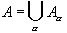#Interactive Real Analysis

## 1. Sets and Relations

### 1.3. Equivalence Relations and Classes

Just as there were different classes of functions (bijections, injections, and surjections), there are also special classes of relations. One of the most useful kind of relation (besides functions, which of course are also relations) are those called equivalence relations.

 Definition 1.3.1: Equivalence Relation Let S be a set and r a relation between S and itself. We call r an equivalence relation on S if r has the following three properties: Reflexivity: Every element of S is related to itself Symmetry: If s is related to t then t is related to s Transitivity: If s is related to t and t is related to u, then s is related to u.
 Examples 1.3.2: Let A = {1, 2, 3, 4} and B = {a, b, c} and define the following two relations: r : { (a,a), (b,b), (a,b), (b,a) } s : 1 ~ 1, 2 ~ 2, 3 ~ 3, 4 ~ 4, 1 ~ 4, 4 ~ 1, 2 ~ 4, 4 ~ 2Which one is an equivalence relation, if any ?
At first glance equivalence relations seem to be too abstract to be useful. However, just the opposite is the case. Because they are defined in an abstract fashion, equivalent relations can be utilized in many different situations. In fact, they can be used to define such basic objects as the integers, the rational numbers, and the real numbers.

The main result about an equivalence relation on a set A is that it induces a partition of A into disjoint sets. This property is the one that will allow us to define new mathematical objects based on old ones in the next section.

 Theorem 1.3.3: Equivalence Classes Let r be an equivalence relation on a set A. Then A can be written as a union of disjoint setswith the following properties: If a, b are in A then a ~ b if and only if a and b are in the same setThe subsetsare non-empty and pairwise disjoint. The setsare called equivalence classes.
 Example 1.3.4:Consider the set Z of all integers. Define a relation r by saying that x and y are related if their difference y - x is divisible by 2. Then Check that this relation is an equivalence relation Find the two equivalence classes, and name them appropriately. How would you add these equivalence classes, if at all ?What kind of equivalence classes do you get when x and y are defined to be related if their difference is divisible by m ? How could you add those ?
Here is another, more complicated example:
 Example 1.3.5: Consider the set R x R \ {(0,0)} of all points in the plane minus the origin. Define a relation between two points (x,y) and (x', y') by saying that they are related if they are lying on the same straight line passing through the origin. Then:Check that this relation is an equivalence relation and find a graphical representation of all equivalence classes by picking an appropriate member for each class. The space of all equivalence classes obtained under this equivalence relation is called projective space.
More examples for equivalence relations and their resulting classes are given in the next section.